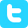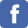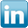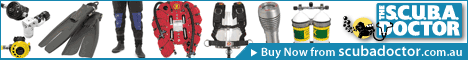search
> contact Lloyd
> Lloyd's blog
> forums# Equivalent Air Depth (EAD) Calculator for Nitrox

Warning: This EAD calculator is for aiding in the calculation of decompression obligations when diving using Nitrox. It is not suitable for calculating narcotic effects and decompression requirements when using Trimix or Heliox.

Disclaimer: I take no responsibility for the accuracy of the calculations and data below. Always verify against other sources.

This calculator can be used to find the equivalent air depth (EAD) in metres and feet of the provided fraction of oxygen (FO2) at various planned diving depths in metres when using Enriched Air Nitrox. Just enter values for "Fraction of Oxygen (FO2)" and "Planned Depth" and click on the "Submit" button.

* Indicates required field
 *Fraction of Oxygen (FO2) e.g. 1=100%, 0.21=21% etc. *Planned Depth e.g. 27 metres

Diving a Fraction of Oxygen (FO2) of .36 or 36% - EAN36 -
(i.e. a Fraction of Nitrogen (FN2) of 0.64 or 64%)
at a Planned Depth of 27 metres (88.6 feet)

## 20.0 metres

in imperial units:

## 66 feet

Disclaimer:
This calculator provides information about gas use in scuba diving. UNDER NO CIRCUMSTANCES is there any implication that any gas mixture is safe or even life supporting at any depth. If you have not recived training in technical scuba diving, Nitrox, Trimix or Heliox you should not attempt to use any of these results. Improper use of gasses in diving can result in injury or DEATH.

## Explanation

We have to know our maximum operating depth (MOD) when we dive using Enriched Air Nitrox (EAN) breathing gas mixtures that contain Nitrogen (N2) and Oxygen (O2) in different proportions to those in air. Such mixtures are known as Enriched Air Nitrox (EAN), or more commonly, Nitrox.

If we have access to Nitrox tables there is not that much more to think about. However, if we only have air tables we have to calculate the equivalent air depth (EAD) in order to be able to determine the approximate decompression requirements for the dive.

The equivalent air depth (EAD) for a given Nitrox mix and planned diving depth, is the depth of a dive when breathing air (i.e. FO2 = .21, O2% = 21%, EAN21) that would have the same partial pressure of nitrogen (ppN2).

## Formulas

To calculate the equivalent air depth (EAD) using Nitrox for a specific FO2 and planned diving depth, the following formulas are used:

#### Metric - metres

EAD (m) = ((Depth + 10) x (FN2 / 0.79)) - 10

In which Depth is the planned diving depth in metres, FN2 is decimal value of the fraction of nitrogen in the mixture and 0.79 is the FN2 in air. For Nitrox, the decimal value of the fraction of nitrogen in the mixture (FN2) is 1 - FO2, the decimal value of the fraction of oxygen in the mixture. For example, if a gas contains 36% oxygen and the planned depth is 27 metres,
the EAD (m) is [(27 + 10) x ((1 - .36) / 0.79)] - 10) = 20 metres.

#### Imperial - feet

EAD (ft) = ((Depth + 33) x (FN2 / 0.79)) - 33

In which Depth is the planned diving depth in feet, FN2 is decimal value of the fraction of nitrogen in the mixture and 0.79 is the FN2 in air. For Nitrox, the decimal value of the fraction of nitrogen in the mixture (FN2) is 1 - FO2, the decimal value of the fraction of oxygen in the mixture. For example, if a gas contains 36% oxygen and the planned depth is 89 feet,
the EAD (ft) is [(89 + 33) x ((1 - .36) / 0.79)] - 33) = 66 feet.

[ previous | up | next ]